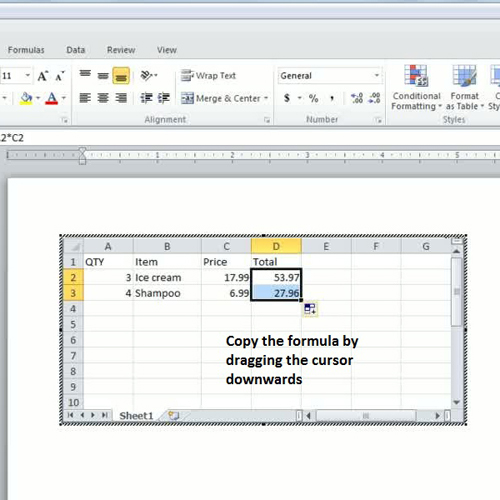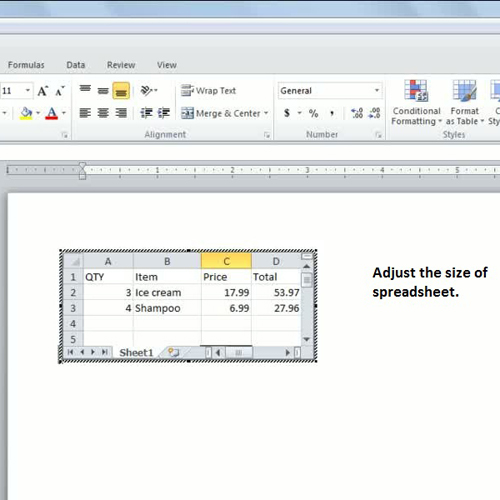## How to insert an Excel spreadsheet in Microsoft Word 2010

If you want to use an excel spreadsheet, you can simply insert excel spreadsheet from a table in a document. Insert spreadsheet in Word so that you can perform all the actions that you’d otherwise perform in Excel. You can do calculations with your data by inserting different formulas and arrange the data in a spreadsheet table.
In this tutorial, you will learn how to insert an Excel spreadsheet in word.

Step # 1 – Insert a spreadsheet

In order to insert spreadsheet in Word, go to the “insert” tab and click on the Table dropdown arrow. Over there, click on the option titled ‘Excel Spreadsheet’. Once done, you will have the spreadsheet will be added to your Word document. Now start typing in the data in separate columns and rows of the spreadsheet.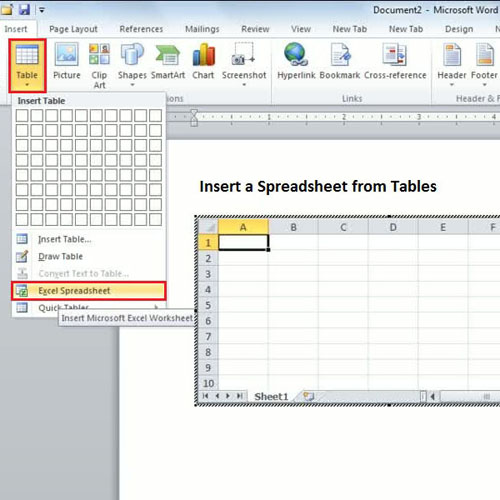Step # 2 – Type data in spreadsheet

The next step is to type data in spreadsheet. In the rows, we are going to enter QTY, Item, Price and Total. To add a little more space in the second column, bring the cursor in between the two columns B and C after which the double arrow will appear; drag it towards right to create more space.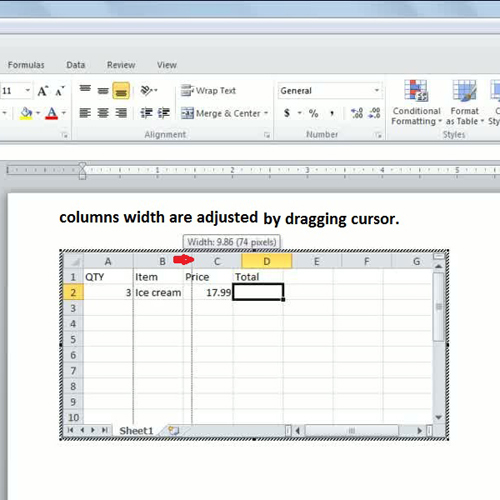Step # 3 – Apply a formula

Since we want to calculate the total price, we will do so by inserting a simple formula. In order to calculate the total price of an ice cream in this tutorial, we entered ‘=A2*C2’. Press the ‘enter’ key and tt will automatically calculate the total price.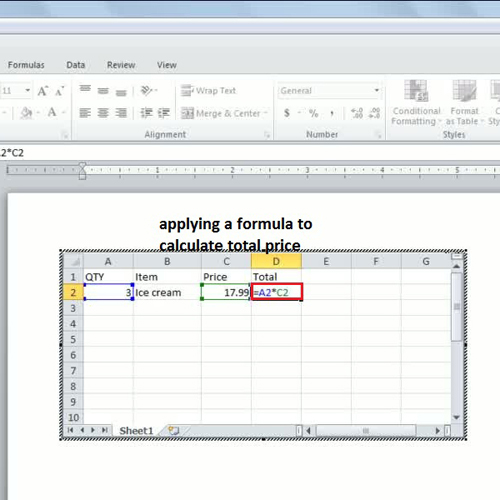Step # 4 – Copy formula

When you want to apply the same function for the next row, you can do so by copying the formula. In order to do so, click on the formula and the cursor will turn into a cross or plus sign; drag it downwards to the row below.
Once done, the total price will be calculated with the help of the formula.